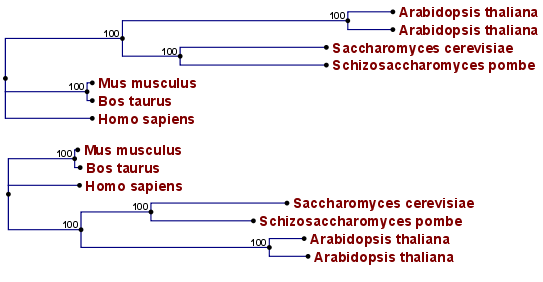## Reconstructing phylogenies from molecular data

Traditionally, phylogenies have been constructed from morphological data, but following the growth of genetic information it has become common practice to construct phylogenies based on molecular data, known as molecular phylogeny. The data is most commonly represented in the form of DNA or protein sequences, but can also be in the form of e.g. restriction fragment length polymorphism (RFLP).

Methods for constructing molecular phylogenies can be distance based or character based.

Distance based methods
Two common algorithms, both based on pairwise distances, are the UPGMA and the Neighbor Joining algorithms. Thus, the first step in these analyses is to compute a matrix of pairwise distances between OTUs from their sequence differences. To correct for multiple substitutions it is common to use distances corrected by a model of molecular evolution such as the Jukes-Cantor model [Jukes and Cantor, 1969].

UPGMA. A simple but popular clustering algorithm for distance data is Unweighted Pair Group Method using Arithmetic averages (UPGMA) ([Michener and Sokal, 1957], [Sneath and Sokal, 1973]). This method works by initially having all sequences in separate clusters and continuously joining these. The tree is constructed by considering all initial clusters as leaf nodes in the tree, and each time two clusters are joined, a node is added to the tree as the parent of the two chosen nodes. The clusters to be joined are chosen as those with minimal pairwise distance. The branch lengths are set corresponding to the distance between clusters, which is calculated as the average distance between pairs of sequences in each cluster.

The algorithm assumes that the distance data has the so-called molecular clock property i.e. the divergence of sequences occur at the same constant rate at all parts of the tree. This means that the leaves of UPGMA trees all line up at the extant sequences and that a root is estimated as part of the procedure.Figure 21.8: Algorithm choices for phylogenetic inference. The bottom shows a tree found by the neighbor joining algorithm, while the top shows a tree found by the UPGMA algorithm. The latter algorithm assumes that the evolution occurs at a constant rate in different lineages.

Neighbor Joining. The neighbor joining algorithm,[Saitou and Nei, 1987], on the other hand, builds a tree where the evolutionary rates are free to differ in different lineages, i.e., the tree does not have a particular root. Some programs always draw trees with roots for practical reasons, but for neighbor joining trees, no particular biological hypothesis is postulated by the placement of the root. The method works very much like UPGMA. The main difference is that instead of using pairwise distance, this method subtracts the distance to all other nodes from the pairwise distance. This is done to take care of situations where the two closest nodes are not neighbors in the "real" tree. The neighbor join algorithm is generally considered to be fairly good and is widely used. Algorithms that improves its cubic time performance exist. The improvement is only significant for quite large datasets.

Character based methods. Whereas the distance based methods compress all sequence information into a single number, the character based methods attempt to infer the phylogeny based on all the individual characters (nucleotides or amino acids).

Parsimony. In parsimony based methods a number of sites are defined which are informative about the topology of the tree. Based on these, the best topology is found by minimizing the number of substitutions needed to explain the informative sites. Parsimony methods are not based on explicit evolutionary models.

Maximum Likelihood. Maximum likelihood and Bayesian methods (see below) are probabilistic methods of inference. Both have the pleasing properties of using explicit models of molecular evolution and allowing for rigorous statistical inference. However, both approaches are very computer intensive.

A stochastic model of molecular evolution is used to assign a probability (likelihood) to each phylogeny, given the sequence data of the OTUs. Maximum likelihood inference [Felsenstein, 1981] then consists of finding the tree which assign the highest probability to the data.

Bayesian inference. The objective of Bayesian phylogenetic inference is not to infer a single "correct" phylogeny, but rather to obtain the full posterior probability distribution of all possible phylogenies. This is obtained by combining the likelihood and the prior probability distribution of evolutionary parameters. The vast number of possible trees means that bayesian phylogenetics must be performed by approximative Monte Carlo based methods.[Larget and Simon, 1999], [Yang and Rannala, 1997].